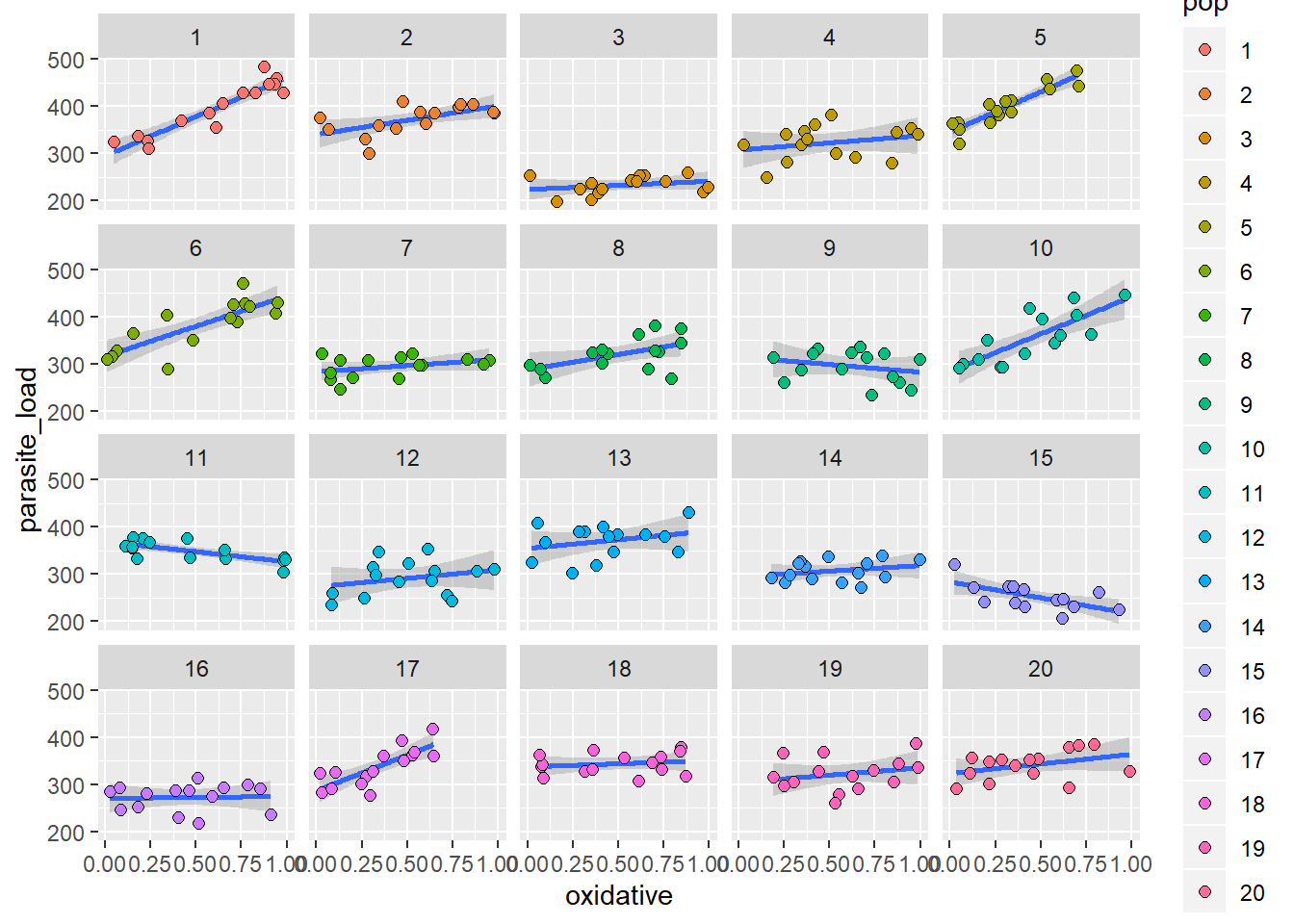Peril 4a: Effect of predictor varies among random effect categories

This is the example where we simulate data on the relationship between oxidative stress and parasites in amphibians and fit random slopes models.

library(MASS)
library(ggplot2)
library(lme4)

Define a function that simulates the data to use in the model

The key parameters for the dataset generated are defined within this function:

Number of populations (npops) = 20 Number of measurements per population (nmeasurements) = 15 oxidative = values of the predictor variable (a measure of oxdiative stress) Hyperparameters for random effects: intercept.mean = 300 intercept.sd = 30 slope.mean = 50 slope.sd = 50 intercept_slope_cov = 50

random_slope_data<-function(){
npops<-20
nmeasurements<-15
n<-nmeasurements*npops

#Population ID
pop_id<-gl(n=npops,k=nmeasurements)

#Random Treatment Assignment
treatment<-sample(rep(c("Control","Treatment"),each=npops/2))
treatment_expand<-treatment[pop_id]

#Oxidative Stress Predictor
oxidative<-runif(n,0,1)

Xmat <- model.matrix(~pop_id*oxidative-1-oxidative)

#Random Effects Parameters
intercept.mean<-300
intercept.sd<-30
slope.mean<-50
slope.sd<-50
intercept_slope_cov<-50

mu.vector <- c(intercept.mean, slope.mean)
var.cova.matrix <- matrix(c(intercept.sd^2,intercept_slope_cov,
intercept_slope_cov, slope.sd^2),2,2)

effects <- mvrnorm(n = npops, mu = mu.vector, Sigma = var.cova.matrix)

intercept.effects <- effects[,1]
slope.effects <- effects[,2]
all.effects <- c(intercept.effects, slope.effects) #Put them all together

lin.pred <- Xmat[,] %*% all.effects   #Value of lin.predictor
eps <- rnorm(n = n, mean = 0, sd = 30)    #residuals
parasites <- lin.pred + eps           #response = lin.pred + residual

#Assemble Data Frame

return(alldata)
} #function end

Here we test an example of the data generation process and plot it

#Generate a Dataset and Inspect
rep1<-random_slope_data()
## 1      354.7967 Treatment   1 0.60993467
## 2      326.5259 Treatment   1 0.23369469
## 3      460.4845 Treatment   1 0.94308233
## 4      325.2640 Treatment   1 0.04936098
## 5      428.9941 Treatment   1 0.82716510
## 6      448.0645 Treatment   1 0.93437084
#Plot Data - Separate Facet for Each Population Showing Relationship (or lack thereof) between oxidative stress and parasite burden
facet_wrap(.~pop) +
geom_smooth(method="lm") +
geom_point(size=2,shape=21,aes(fill=pop))Here we do the set-up necessary for the simulations

Set seed for reproducibility, define the number of simulations conducted and create the emptry matrix to store results.

There is also the possibility to change the outcome of warnings from lme4 here

#Random Number Seed For Reproducibility
set.seed(123456)

#How Many Iterations
nsims<-10000

#Blank Results Matrix With Column Names
resultsmat<-matrix(nrow=nsims,ncol=4)
colnames(resultsmat)<-c("model1_treatment_main_effect","model1_treatment_interaction","model2_treatment_main_effect","model2_treatment_interaction")

#Library for Running Models
#options(warn=-1)

Now we conduct the simulations

NB. We are suppressing the warning messages from model fitting from being printed here for presentation purposes. We would recommend re-running after deleting warning=FALSE and message=FALSE from the line below this to see what warnings arise and when they do if you are interested.

for(k in 1:nsims){

#Generate Data
rep1<-random_slope_data()
#Fit RANDOM INTERCEPT Model incl interaction between oxidative stress and random 'treatment' variable
m1<-lmer(parasite_load ~ oxidative * treatment + (1|pop),data=rep1)
#Generate Confidence Intervals for Effects
m1conf<-confint(m1,method="Wald")
#Assess Whether 95% Confidence Intervals cross zero
resultsmat[k,1]<-m1conf[5,1]<0 & m1conf[5,2]>0
resultsmat[k,2]<-m1conf[6,1]<0 & m1conf[6,2]>0

#Fit RANDOM SLOPE Model incl interaction between oxidative stress and random 'treatment' variable
#May throw singularity errors in a small proportion of cases.
m2<-lmer(parasite_load ~ oxidative * treatment + (oxidative|pop),data=rep1)
m2conf<-confint(m2,method="Wald")
resultsmat[k,3]<-m2conf[7,1]<0 & m2conf[7,2]>0
resultsmat[k,4]<-m2conf[8,1]<0 & m2conf[8,2]>0

} #k loop end

Calculate type I error rates for:

Column 1 - main effect of treatment in random intercepts model

Column 2 - interaction between treatment and oxidative stress in random intercepts model

Column 3 - main effect of treatment in random slopes model

Column 4 - interaction between treatment and oxidative stress in random slopes model

1-apply(resultsmat,2,mean,na.rm=T)
## model1_treatment_main_effect model1_treatment_interaction
##                       0.0198                       0.3055
## model2_treatment_main_effect model2_treatment_interaction
##                       0.0701                       0.0672